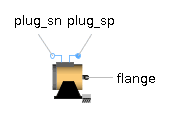Convection - MapleSim Help

Convection

Lumped thermal element for heat convectionDescription The Convection component models a linear heat convection between solid geometries and fluid flow over the solid using a convective thermal conductance controlled by an input signal.Equations ${Q}_{\mathrm{flow}}={Q}_{{\mathrm{flow}}_{\mathrm{solid}}}=-{Q}_{{\mathrm{flow}}_{\mathrm{fluid}}}={G}_{c}\Delta T$ $\Delta T={T}_{\mathrm{solid}}-{T}_{\mathrm{fluid}}$Variables

 Name Units Description Modelica ID ${Q}_{\mathrm{flow}}$ $W$ Heat flow rate from solid -> fluid Q_flow $\Delta T$ $K$ = solid.T - fluid.T dTConnections

 Name Description Modelica ID ${G}_{c}$ Real input; convective thermal conductance in $\frac{W}{K}$ Gc $\mathrm{solid}$ HeatPort for solid solid $\mathrm{fluid}$ HeatPort for fluid fluidModelica Standard Library The component described in this topic is from the Modelica Standard Library. To view the original documentation, which includes author and copyright information, click here.